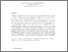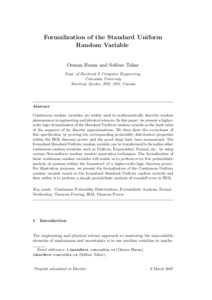Title:

# Formalization of the Standard Uniform random variable

Hasan, Osman and Tahar, Sofiène (2007) Formalization of the Standard Uniform random variable. Theoretical Computer Science, 382 (1). pp. 71-83. ISSN 03043975Preview Text (application/pdf) formalization_of_the_standard_uniform_random_variable.pdf - Accepted Version 220kB

Official URL: http://dx.doi.org/10.1016/j.tcs.2007.05.009

## Abstract

Continuous randomvariables are widely used to mathematically describe random phenomena in engineering and the physical sciences. In this paper, we present a higher-order logic formalization of the StandardUniformrandomvariable as the limit value of the sequence of its discrete approximations. We then show the correctness of this specification by proving the corresponding probability distribution properties within the HOL theorem prover, summarizing the proof steps. The formalized StandardUniformrandomvariable can be transformed to formalize other continuous randomvariables, such as Uniform, Exponential, Normal, etc., by using various non-uniformrandom number generation techniques. The formalization of these continuous randomvariables will enable us to perform an error free probabilistic analysis of systems within the framework of a higher-order-logic (HOL) theorem prover. For illustration purposes, we present the formalization of the Continuous Uniformrandomvariable based on the formalized StandardUniformrandomvariable, and then utilize it to perform a simple probabilistic analysis of roundoff error in HOL.

Divisions: Concordia University > Gina Cody School of Engineering and Computer Science > Electrical and Computer Engineering Article Yes Hasan, Osman and Tahar, Sofiène Theoretical Computer Science 2007 10.1016/j.tcs.2007.05.009 974501 ANDREA MURRAY 31 Jul 2012 20:08 18 Jan 2018 17:38
All items in Spectrum are protected by copyright, with all rights reserved. The use of items is governed by Spectrum's terms of access.

Repository Staff Only: item control pageResearch related to the current document (at the CORE website)
Back to top